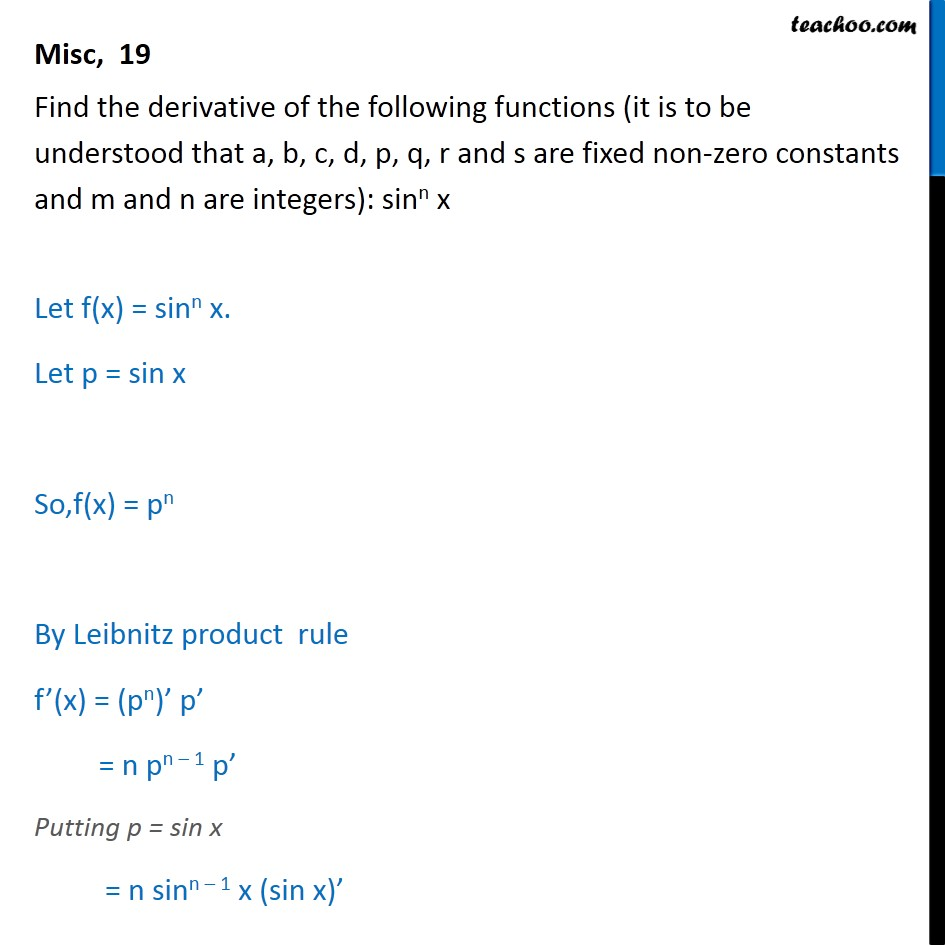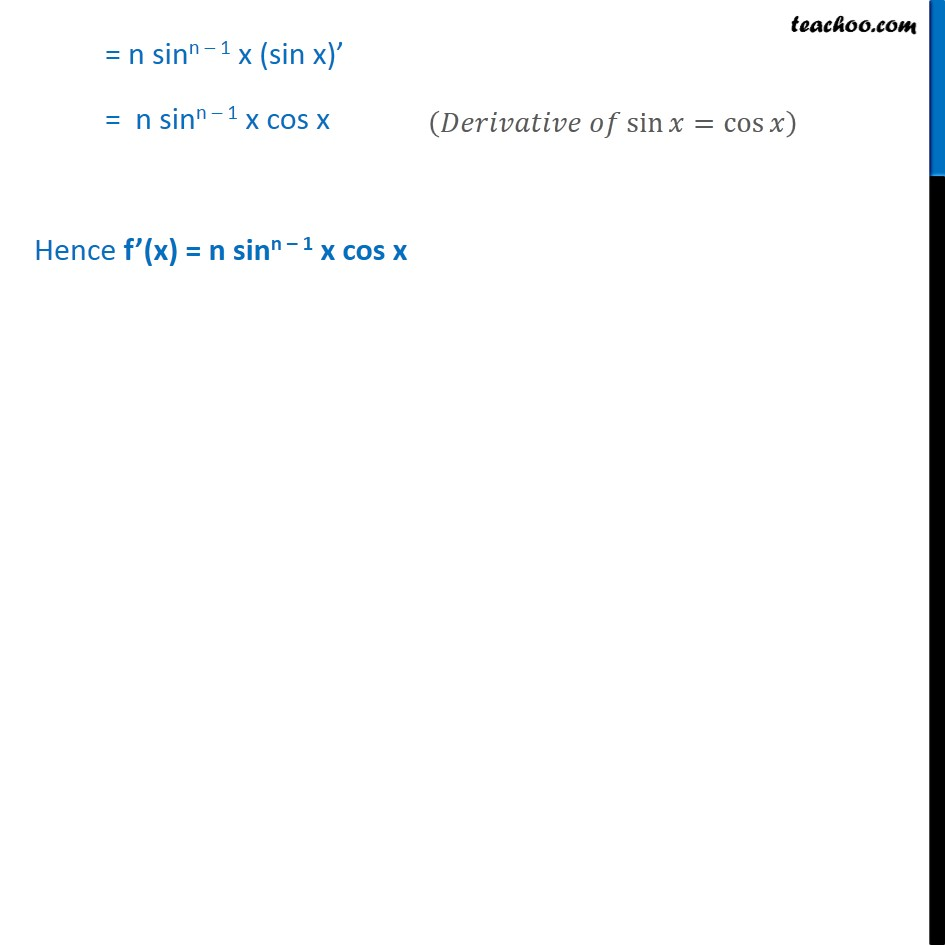Miscellaneous

Chapter 12 Class 11 Limits and Derivatives
Serial order wiseLearn in your speed, with individual attention - Teachoo Maths 1-on-1 Class

### Transcript

Misc 19 Find the derivative of the following functions (it is to be understood that a, b, c, d, p, q, r and s are fixed non-zero constants and m and n are integers): sinn x Let f(x) = sinn x. Let p = sin x So,f(x) = pn By Leibnitz product rule f’(x) = (pn)’ p’ = n pn – 1 p’ Putting p = sin x = n sinn – 1 x (sin x)’ = n sinn – 1 x (sin x)’ = n sinn – 1 x cos x Hence f’(x) = n sinn – 1 x cos x